Get Free NCERT Solutions for Class 10 Maths Chapter 8 Ex 8.3 Introduction to Trigonometry Class 10 Maths NCERT Solutions are extremely helpful while doing homework. Exercise 8.3 Class 10 Maths NCERT Solutions were prepared by Experienced LearnCBSE.in Teachers. Detailed answers of all the questions in Chapter 8 Maths Class 10 Coordinate Geometry Exercise 8.3 Provided in NCERT Textbook.

Topics and Sub Topics in Class 10 Maths Chapter 8 Introduction to Trigonometry:

 Section Name Topic Name 8 Introduction to Trigonometry 8.1 Introduction 8.2 Trigonometric Ratios 8.3 Trigonometric Ratios Of Some Specific Angles 8.4 Trigonometric Ratios Of Complementary Angles 8.5 Trigonometric Identities 8.6 Summary

You can also download the free PDF of Chapter 8 Ex 8.3 Coordinate Geometry NCERT Solutions or save the solution images and take the print out to keep it handy for your exam preparation.

 Board CBSE Textbook NCERT Class Class 10 Subject Maths Chapter Chapter 8 Chapter Name Introduction to Trigonometry Exercise Ex 8.3 Number of Questions Solved 7 Category NCERT Solutions

## NCERT Solutions For Class 10 Maths Chapter 8 Introduction to Trigonometry Ex 8.3

NCERT Solutions for Class 10 Maths Chapter 8 Introduction to Trigonometry Ex 8.3 are part of NCERT Solutions for Class 10 Maths. Here we have given NCERT Solutions for Class 10 Maths Chapter 8 Introduction to Trigonometry Ex 8.3.

Ex 8.3 Class 10 Maths Question 1.
Evaluate:Solution:Ex 8.3 Class 10 Maths Question 2.
Show that:
(i) tan 48° tan 23° tan 42° tan 67° = 1
(ii) cos 38° cos 52° – sin 38° sin 52° = 0
Solution: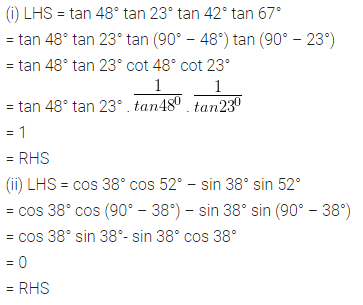Download NCERT Solutions For Class 10 Maths Chapter 8 Introduction to Trigonometry PDF

Ex 8.3 Class 10 Maths Question 3.
If tan 2A = cot (A – 18°), where 2A is an acute angle, find the value of A.
Solution: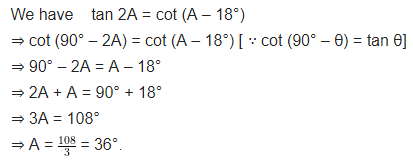Ex 8.3 Class 10 Maths Question 4.
If tan A = cot B, prove that A + B = 90°.
Solution: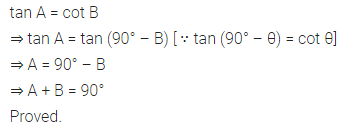Ex 8.3 Class 10 Maths Question 5.
If sec 4A = cosec (A – 20°), where 4A is an acute angle, find the value of A.
Solution: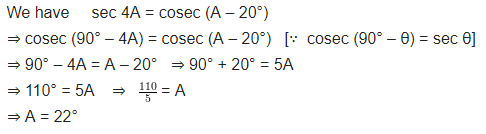Ex 8.3 Class 10 Maths Question 6.
If A, B and C are interior angles of a triangle ABC, then show that: sin ($$\frac { B+C }{ 2 }$$) = cos $$\frac { A }{ 2 }$$
Solution:Ex 8.3 Class 10 Maths Question 7.
Express sin 61° + cos 75° in terms of trigonometric ratios of angles between 0° and 45°.
Solution:### NCERT Solutions for Class 10 Maths Chapter 8 Introduction to Trigonometry (Hindi Medium) Ex 8.3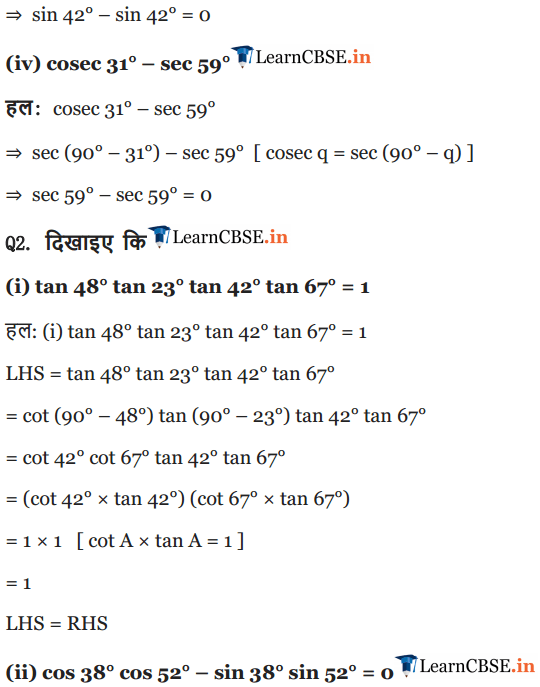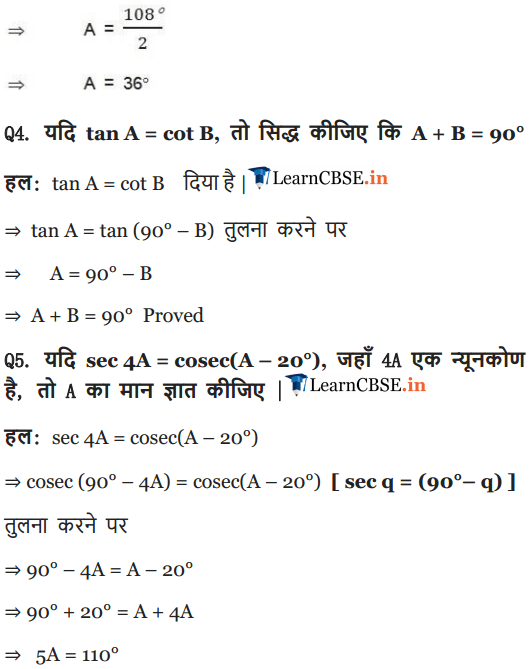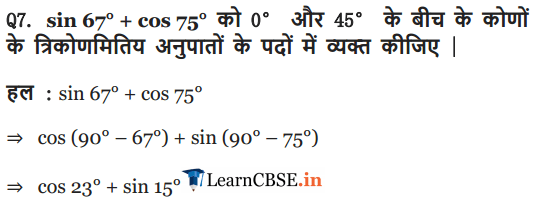We hope the NCERT Solutions for Class 10 Maths Chapter 8 Introduction to Trigonometry Ex 8.3, help you. If you have any query regarding NCERT Solutions for Class 10 Maths Chapter 8 Introduction to Trigonometry Exercise 8.3, drop a comment below and we will get back to you at the earliest.